# Writing a mixed number and an improper fraction for a shaded region Online Quiz

#### Complete Python Prime Pack

9 Courses     2 eBooks

#### Artificial Intelligence & Machine Learning Prime Pack

6 Courses     1 eBooks

#### Java Prime Pack

9 Courses     2 eBooks

Following quiz provides Multiple Choice Questions (MCQs) related to Writing a mixed number and an improper fraction for a shaded region. You will have to read all the given answers and click over the correct answer. If you are not sure about the answer then you can check the answer using Show Answer button. You can use Next Quiz button to check new set of questions in the quiz.Q 1 - Write a mixed number and an improper fraction for the shaded region given below.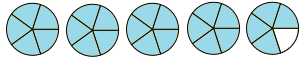### Explanation

Step 1:

There are four whole units and a fractional part $\frac{4}{5}$.

Step 2:

So, the shaded region is represented by mixed number $4\frac{4}{5}$

Step 3:

$4\frac{4}{5} = \frac{\left ( 4 \times 5 + 4 \right )}{5} = \frac{24}{5}$, the improper fraction

Q 2 - Write a mixed number and an improper fraction for the shaded region given below.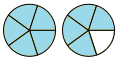### Explanation

Step 1:

There is one whole unit and a fractional part $\frac{4}{5}$.

Step 2:

So, the shaded region is represented by mixed number $1\frac{4}{5}$

Step 3:

$1\frac{4}{5} = \frac{\left ( 1 \times 5 + 4 \right )}{5} = \frac{9}{5}$, the improper fraction

Q 3 - Write a mixed number and an improper fraction for the shaded region given below.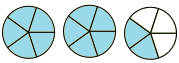### Explanation

Step 1:

There are two whole units and a fractional part $\frac{2}{5}$.

Step 2:

So, the shaded region is represented by mixed number $2\frac{2}{5}$

Step 3:

$2\frac{2}{5} = \frac{\left ( 2 \times 5 + 2 \right )}{5} = \frac{12}{5}$, the improper fraction

Q 4 - Write a mixed number and an improper fraction for the shaded region given below.### Explanation

Step 1:

There are two whole units and a fractional part $\frac{4}{5}$.

Step 2:

So, the shaded region is represented by mixed number $2\frac{4}{5}$.

Step 3:

$2\frac{4}{5} = \frac{\left ( 2 \times 5 + 4 \right )}{5} = \frac{14}{5}$, the improper fraction

Q 5 - Write a mixed number and an improper fraction for the shaded region given below.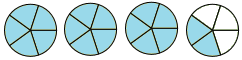### Explanation

Step 1:

There are three whole units and a fractional part $\frac{2}{5}$.

Step 2:

So, the shaded region is represented by mixed number $3\frac{2}{5}$.

Step 3:

$3\frac{2}{5} = \frac{\left ( 3 \times 5 + 2 \right )}{5} = \frac{17}{5}$, the improper fraction

Q 6 - Write a mixed number and an improper fraction for the shaded region given below.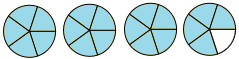### Explanation

Step 1:

There are three whole units and a fractional part $\frac{4}{5}$.

Step 2:

So, the shaded region is represented by mixed number $3\frac{4}{5}$.

Step 3:

$3\frac{4}{5} = \frac{\left ( 3 \times 5 + 4 \right )}{5} = \frac{19}{5}$, the improper fraction

Q 7 - Write a mixed number and an improper fraction for the shaded region given below.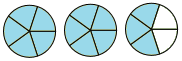### Explanation

Step 1:

There are two whole units and a fractional part $\frac{3}{5}$.

Step 2:

So, the shaded region is represented by mixed number $2\frac{3}{5}$.

Step 3:

$2\frac{3}{5} = \frac{\left ( 2 \times 5 + 3 \right )}{5} = \frac{13}{5}$, the improper fraction

Q 8 - Write a mixed number and an improper fraction for the shaded region given below.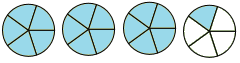### Explanation

Step 1:

There are three whole units and a fractional part $\frac{1}{5}$.

Step 2:

So, the shaded region is represented by mixed number $3\frac{1}{5}$.

Step 3:

$3\frac{1}{5} = \frac{\left ( 3 \times 5 + 1 \right )}{5} = \frac{16}{5}$, the improper fraction

Q 9 - Write a mixed number and an improper fraction for the shaded region given below.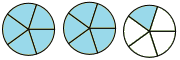### Explanation

Step 1:

There are two whole units and a fractional part $\frac{1}{5}$.

Step 2:

So, the shaded region is represented by mixed number $2\frac{1}{5}$.

Step 3:

$2\frac{1}{5} = \frac{\left ( 2 \times 5 + 1 \right )}{5} = \frac{11}{5}$, the improper fraction

Q 10 - Write a mixed number and an improper fraction for the shaded region given below.### Explanation

Step 1:

There are four whole units and a fractional part $\frac{1}{5}$.

Step 2:

So, the shaded region is represented by mixed number $4\frac{1}{5}$.

Step 3:

$4\frac{1}{5} = \frac{\left ( 4 \times 5 + 1 \right )}{5} = \frac{21}{5}$, the improper fraction